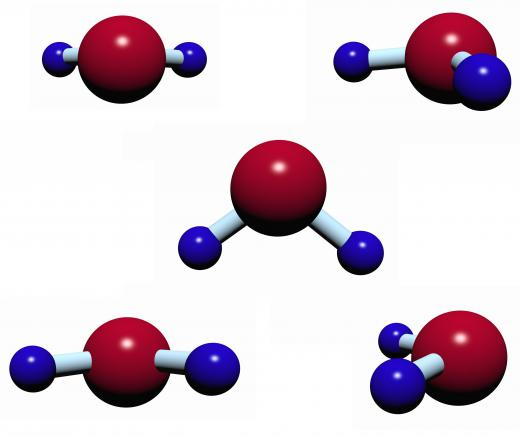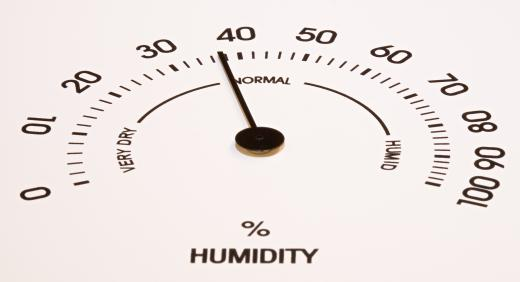# What Factors Affect Air Density?

C. Putnam
C. PutnamThe air density at the top of Mount Everest is much lower than at sea level.

Density is the measurement of the mass of a material in a given volume of space. For a solid, density remains constant even as its environment changes. The density of a gold bar is the same at sea level, under the ocean, and at the top of Mount Everest. For a gas such as air, the density is impacted by changes in pressure, temperature, and humidity. Changes in air density can modify how easily an object moves and even can cause health problems.Number of water molecules has a minor effect on air density,.

Air is a mixture of nitrogen, oxygen, and other trace gases. The molecules of these gases typically move rapidly, bouncing off of each other. When the environment is a constant without changes in temperature, pressure, or humidity, the mass of air in a given volume of space will remain stable. The value of air density, generally given in kilograms per cubic meter, is calculated rather than directly measured.

Increases or decreases in air pressure can impact the density of air. The two major reasons for changes in air pressure are altitude and weather systems. Of these, altitude is the most significant.The density of solids remains the same at all elevations.

For the most part, air becomes denser as air pressure increases. The additional pressure forces the air molecules closer together, resulting in more mass in the given volume. As the altitude increases, the air pressure decreases: there is less pressure pushing the air molecules together. This decrease in air density means that a mountain climber at high altitudes gets less oxygen when he takes a breath. The lungs contain the same volume but the lower air density means that there is less oxygen in the space.Air pressure decreases at high altitudes.

Temperature is the other major factor that can have an effect on the density of air. When the temperature increases, air molecules move faster and bounce further apart; therefore, higher temperatures result in lower air density. When air is denser, it creates drag on objects moving through it. For example, a golf ball hit on a hot day at a golf course on the top of a mountain will go further than one hit on a cold day at sea level. The high temperature and lower air pressure found at high altitudes combine to lower the air density.At high humidity, air density decreases due to the reduced mass in a given volume.

Although a relatively minor effect, the humidity or amount of moisture in the atmosphere also can change the density of air. Surprisingly, the more moisture there is in the air results in lower air density. Since the number of molecules is constant, when the number of water molecules increase the number of nitrogen and oxygen molecules must decrease. The molecular weight of water is much lower than that of dry air whose weight is dominated by nitrogen and oxygen. At high humidity, therefore, the air density decreases due to the reduced mass in a given volume.anon350065

Post 2 is incorrect. Density is not mass times volume. It is mass divided by volume.

mitch8

Well I'm still wondering about the golfer's question. If it is more humid, with more water molecules in the air, is there more drag on a golf ball or less?

anon269844

I can understand that as the humidity rises, there is less room for nitrogen and oxygen molecules in a given area and therefore the density is lower.

A question my friends and I have though, pertains to our golfing. If the humidity is high, the air is less dense - does this mean a golf ball would travel farther in higher humidity (given the same amount force)? Or would the water molecules slow the ball down more?

DentalFloss

@anon66087- Density is, in really basic terms, mass times volume. In other words, how much something weighs multiplied by how much space it occupies. In things air density, this matters because it is one of the steps towards scientists assessing the chemicals in that air, including toxins and imbalances, like too much carbon dioxide.

anon66087

what is density about?